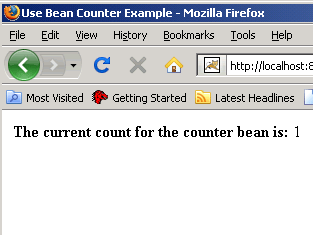Tutorials

# Using Bean Counter in JSP

In this section you will learn how the counter bean can be used in jsp. As you all know a counter increments the value by one. Here, we will use bean with a jsp.

In this section you will learn how the counter bean can be used in jsp. As you all know a counter increments the value by one. Here, we will use bean with a jsp.

Using Bean Counter in JSP

In this section you will learn how the counter bean can be used in jsp. As you all know a counter increments the value by one. Here, we will use bean with a jsp.

Here is an example which explains the purpose.

Code of counter.jsp:

 <%@ page language="java" %> Use Bean Counter Example
The current count for the counter bean is: <%=counter.getCoun() %>

Code of CounterBean.java:

 ``` package form; public class CounterBean implements java.io.Serializable{ int coun = 0; public CounterBean() { } public int getCoun() { coun++; return this.coun;   } public void setCoun(int coun) { this.coun = coun;   } }```

In the above example, A jsp page sets the session by scope="session" and calls the Bean by class="form.CounterBean". A variable 'coun' is initialized to 0 which will return the coun++. The method <%=counter.getCoun() %> is then called by the jsp and the counter value will get incremented by one till the session continues. After the compilation, run the tomcat. Type the URL on the browser.

Output will be displayed as:After refreshing the browser, the output will be displayed as: